# Convert array string to integer

Hello.
I am with an activity, in which I extract a text, cut it and obtain a number that I need to convert to integer format, to be able to compare it later, but I cannot convert it, since it is in an array.
I show you the process.

Array_int.xaml (8,0 KB)

Example imagen:
Array_int2.xaml (7,4 KB)

Hello @Aguirre ,

You might try an assign like this:

``````intArray = Array.ConvertAll(New_Text, Function(str) Int32.Parse(str))
``````

Hope it helps!
Best regards,
Marius

1 Like

Hi @Aguirre

You can try this

Cint(Trim(New_text(1)))

Regards
Sudharsan

Hello, I have tried as you tell me, but it gives me an error, would it be like this?
ERro:
A value of type ‘-1 dimensional array of integer’ cannot be converted to integer

@Aguirre

Please use `int.TryParse` instead of `Int32.Parse`.
It seems that not all your data split by “:” can be an integer.

Best regards,
Marius

you can try with LINQ, using Select:

Input = Array of strings (Result of the Split assign)
Output = Array of Int32

``````var_arr_Strings.Select(function(x) CInt(x)).ToArray
``````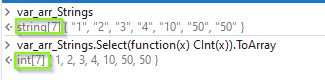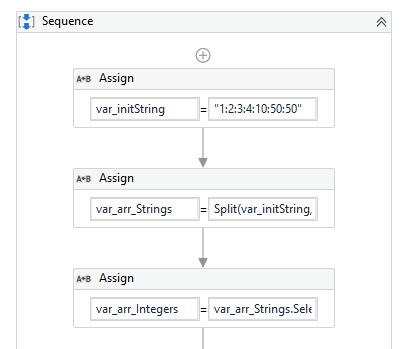Edit:
In case there is possibility of having strings in between, for example, taking this string as original input:

``````"1:2:3:4:10:50:50:abc"
``````

You can add a .Where to the LINQ, to make sure it only returns the Int32 results.

• Simply change the code above, by this code:
``````var_arr_Strings.Where(function(y) IsNumeric(y)).Select(function(x) CInt(x)).ToArray
``````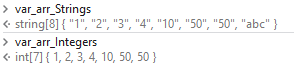1 Like

Hello gives error:
Option Strict does not allow the implicit conversion of integer in string

Hello.
If that’s how I put it, it doesn’t work either.

Hi, I think I’m not doing something right.
ERROR:

Assign: Conversion from string “Number of items found” to type ‘Integer’ is not valid.

Yep, one of the values is text, which contains the string: “Número de elementos encontrados”

• To avoid getting this error, please try to use the 2nd LINQ expression I posted:
``````var_arr_Strings.Where(function(y) IsNumeric(y)).Select(function(x) CInt(x)).ToArray
``````

I think I’m not putting something right, since it keeps giving an error and it’s a simple example.

“var_arr_Strings.Where(Function(y) IsNumeric(y)).Select(Function(x) CInt(x)).ToArray”.
An instance member of a class cannot be referenced from a shared method or shared member initializer without an explicit instance of the class.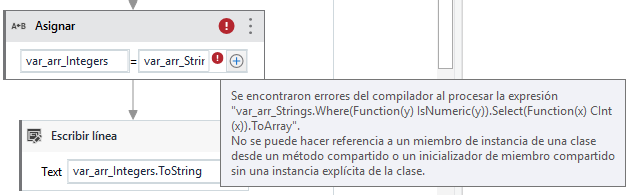Hello, I publish my example, to see if it is easier to find the solution
Array_int.xaml (8,0 KB)

At times, we would need to access `IsNumeric` method on the object, hence `IsNumeric(y)` should be changed to `y.IsNumeric` and Checked.

Please check the following 2 options:

Based on your input from images above, you could use regex to extract the number directly, without the need of arrays:

Option1: Arrays + LINQ
TestLinq.xaml (6.6 KB)

Option2: Regex
Test_Regex.xaml (5.2 KB)

Regex solution in a nutshell: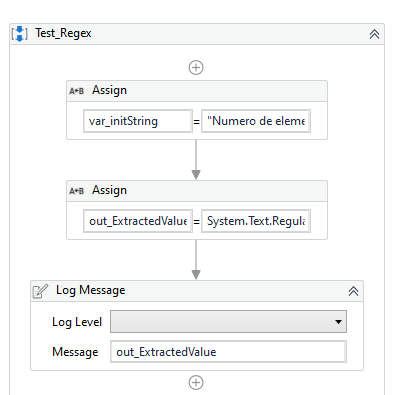out_ExtractedValue = System.Text.RegularExpressions.Regex.Matches(var_initString,“\d”)(0).ToString

1 Like

When comparing the obtained value it does not do it correctly.

Use this in assign
`IntArr = StrArr.Select(Function (p) cint(p)).toArray`

The LINQ solution gives an error before executing it, without touching anything.

out_extractedValue on the examples I sent, was of type string, and you can’t compare “9”<“10” with strings

Try This:

on the if: CInt(out_ExtractedValue) > 10

Hello, would it be as you say?
it gives me an error.

I given:
"CInt(out_ExtractedValue) >“10"”.
Option Strict On does not allow implicit conversion of ‘String’ to